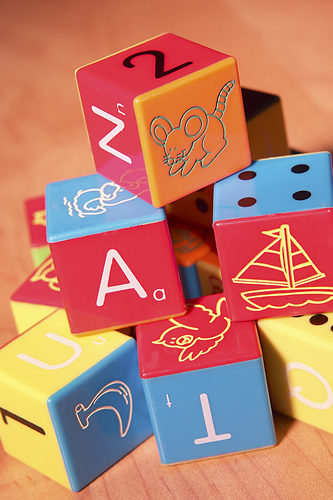• Mathematics:

Operations and processes involved in the solution of a problem or study of some scientific field can be identified as the mathematical process.  Mathematics is a group of related sciences, including algebra, geometry, and calculus, concerned with the study of a number, quantity, size, shape, and space; including their interrelationships by using a specialized notation.

Developmental Spectrum of Mathematical Skills:

8. Problem Solving– Reasoning(word problems w/bar modeling)

7. Concepts & Application(M-CAP)

geometry, measurement, algebra, data, probability, statistics, money, time

graphing, estimation

6. Computation/Operations(+, -, x, ÷)(M-COMP)

sorting, graphing, set relationships, series, estimating

5. Missing Number (MNM)

4. QuantityDiscrimination(QDM)

3. NumberIdentification (NIM)

2. Oral Counting (OCM)

1. Number Sense (OCM, NIM, QDM, MNM)

sequencing, succession,1 to 1 correspondence

(basic level concepts = introduction to number, shape, size, weight, time)